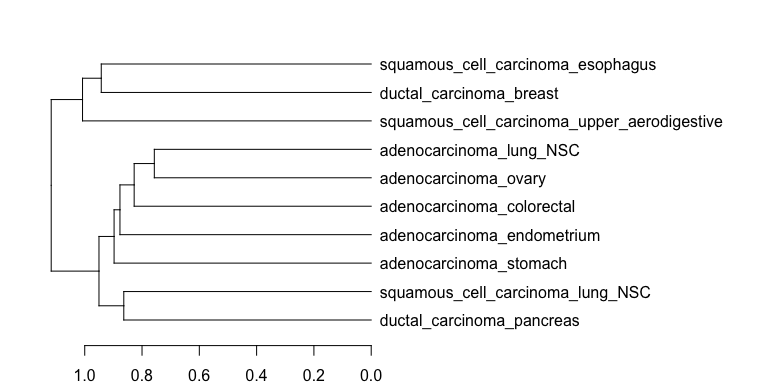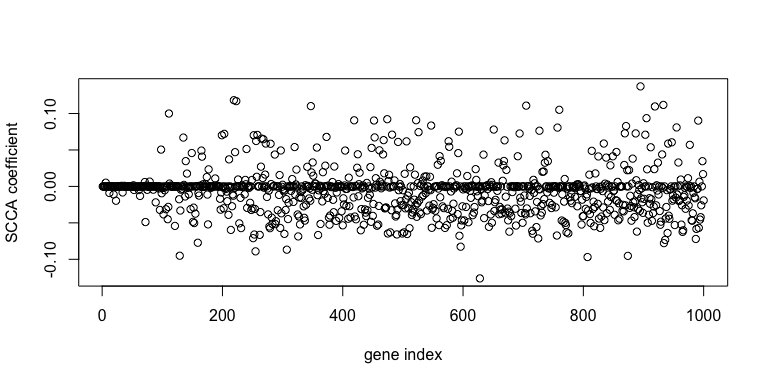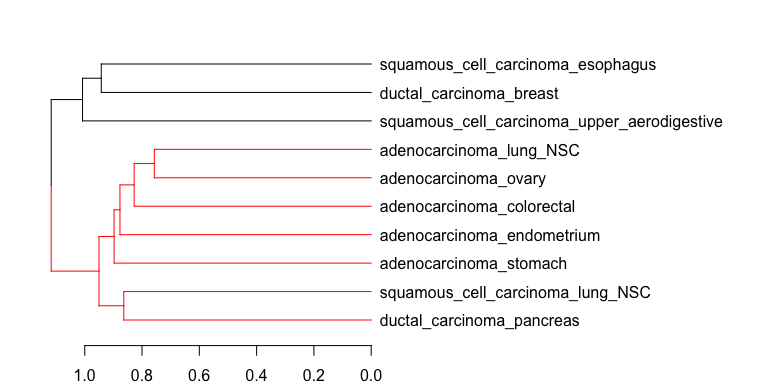# hierBipartite Vignette

## 1. Introduction

Pharmacogenomic studies are often interested in the relationship between a set of genomic features and a set of drug responses, for purposes such as biomarker discovery or building drug-response models. We propose a framework for clustering pre-defined cell line groups in terms of gene-drug relationships, which we refer to as bipartite graph-based hierarchical clustering. This enables applications such as visualization of group similarities or determining which cell line groups to include for downstream analysis.

The $$\texttt{hierBipartite}$$ R package implements the bipartite graph-based hierarchical clustering method detailed in the paper. The “bipartite graph” describes the association relationship between the set of genes and the set of drugs. The method starts by creating a dissimilarity matrix for the provided starting cell line groups by (1) extracting gene-drug association patterns for each group using sparse canonical correlation analysis (SCCA) and (2) using a nuclear norm-based dissimilarity measure to compare groups based on the extracted association patterns. The $$\texttt{hierBipartite}$$ package applies hierarchical clustering to determine the hierarchical relationship between the cell line groups. A few optional procedures are implemented to enhance this framework:

• Subsampling to extract more robust gene-drug association patterns: for a given group of cell lines, SCCA extracts gene-drug association patterns on a subsample of cell lines. The average result from repetition of this procedure yields more robust gene-drug association patterns.
• Permutation test: for two clusters of cell lines merging at a non-leaf node in the dendrogram, determine the p-value of similarity in gene-drug association patterns (smaller values indicates greater significance). These p-values are calculated in a bottom-up fashion, following the merge order in hierarchical clustering. The $$\texttt{hierBipartite}$$ package implements early-stopping, which stops calculating p-values once a p-value exceed a threshold is encountered, for computational efficiency. This is because once cell lines from groups sharing little gene-drug association patterns are pooled together, any further merging with other groups will no longer be meaningful as well.

## 2. Load Data

The $$\texttt{hierBipartite}$$ R package contains a test dataset based on gene expression dataset from the Cancer Cell Line Encyclopedia (CCLE) resource and drug sensitivity dataset from the Cancer Therapeutics Response Portal (CTRP2) resource (Barretina et al. 2012; Seashore-Ludlow et al. 2015). In the paper, this dataset is referred to as “CTRP2”, named after the drug sensitivity resource.

The CTRP2 dataset has been processed exactly as described in the paper, with the exception of selecting the top 1,000 transcripts instead of 5,000 transcripts by correlation with drug sensitivity values. This is to abide by the Bioconductor memory constraint of less than or equal to 5 MB for individual files. However, the purpose of this test dataset is to demonstrate how to use the $$\texttt{hierBipartite}$$ package. For additional details on the CTRP2 dataset, execute ?ctrp2. The data structure of the CTRP2 dataset is as follows:

• $$X \in \mathbb{R}^{n \times p}$$: gene expression measured in $$\log_{2}TPM$$1, for $$n$$ cell lines across $$p$$ genes.
• $$Y \in \mathbb{R}^{n \times q}$$: drug sensitivity measured in terms of area over dose-response curve, for $$n$$ cell lines across $$q$$ drugs.
• groups: List of starting cell line groups. Each group is represented by a vector of row indices for $$X, Y$$.

First load this test dataset and extract the above three components.

library(hierBipartite)
data(ctrp2)

# gene expression
X = ctrp2[["X"]]
# drug sensitivity
Y = ctrp2[["Y"]]
# starting cell line groups
groups = ctrp2[["groups"]]

List the 10 cell line groups present in this dataset. The cell lines are grouped by carcinoma subtype (e.g. adenocarcinoma) and primary site (e.g. lung NSC).

names(groups)
##   "adenocarcinoma_colorectal"                   "adenocarcinoma_endometrium"
##   "adenocarcinoma_lung_NSC"                     "ductal_carcinoma_breast"
##   "ductal_carcinoma_pancreas"                   "squamous_cell_carcinoma_esophagus"
##   "squamous_cell_carcinoma_upper_aerodigestive" "squamous_cell_carcinoma_lung_NSC"

Show each group is represented by vector of row indices in $$X, Y$$

# Row indices of samples in X, Y from adenocarcinoma, lung NSC
groups[["adenocarcinoma_lung_NSC"]]
##    87  89  95 101 103 104 105 106 112 113 117 118 119 121 123 124 125 126 127 132 133 136 139 140 144 146 148 149 150 151
##  158 159 163

## 3. Bipartite Graph-based Hierarchical Clustering

### 3.1 Using $$\texttt{hierBipartite}$$

The bipartite graph-based hierarchical clustering method is applied using the hierBipartite() main function, which takes two data matrices X and Y as input and a groups list indicating how samples are grouped. The link parameter determines the link function to use for hierarchical clustering, which can be one of “ward.D”, “ward.D2”, “single”, “complete”, “average”, “mcquitty”, “median”, or “centroid”. If applying the optional procedures described in the Introduction:

• Subsampling to extract more robust gene-drug association patterns: the parameters are number of subsampling rounds n_subsample and subsampling proportion subsampling_ratio (value in (0, 1]). If the user does not want to run this procedure, then set n_subsample = 1 and subsampling_ratio = 1. A larger n_subsample value results in greater robustness, but also increased runtime. The n_subsample = 100 value is usually sufficient. When the group sizes are small (e.g. < 50), it is recommended to set subsampling_ratio to a larger value, such as subsampling_ratio = 0.90.
• Permutation test: the parameters are number of permutations n_perm and early-stopping threshold p_cutoff. Usually, n_perm values ranging from 100 to 1,000 are sufficient. The permutation test can be toggled on with p.value = TRUE.

Although the subsampling procedures and the permutation tests are computationally intensive, each subsample step or permutation test is independent of each other. Thus, we can parallelize these steps using the parallel package, by toggling parallel = TRUE.

Now we apply hierBipartite() method on the test data with the permutation test, but without performing the subsampling procedure to save runtime. This process could take 1 - 2 hours (for 260 cell lines, 1,000 genes, 133 drugs, and 10 groups).

set.seed(1)
result = hierBipartite(X = X, Y = Y, groups = groups, p.value = TRUE,
n_perm = 100, parallel = TRUE, p_cutoff = 0.1)

### 3.2 Examining Results

The hierBipartite() method outputs a list containing

• hclust: hclust object from hierarchical clustering.
• groupMerges: list of clusters after each merge, in order of merge. Each cluster is indicated by a vector of cell line groups.
• nodePvals: list p-value of each new merge, in order of merge. Only exists if p.value = TRUE.
• D: dissimilarity matrix.

To view the resulting dendrogram

hclustObj = result[["hclustObj"]]
par(mar = c(2,2,2,20))
plot(as.dendrogram(hclustObj), horiz = TRUE)We now illustrate how to answer some common questions about the results after the method is finished. To get the groups in the third merge and corresponding p-value:

print(result$groupMerges[]) ##  "ductal_carcinoma_pancreas" "squamous_cell_carcinoma_lung_NSC" print(result$nodePvals[])
##  0

Suppose we are interested in selecting the expression and drug sensitivity data from cell lines in the third merge for downstream analysis.

groups2rows = function(groups, cluster) {
# Input:
#   groups: a list of starting group membership (e.g. list("1" = c(1,2,3), "2" = c(4,5,6)) means group 1 has
#           samples 1, 2, 3, and group 2 has samples 4, 5, 6.
#   cluster: a vector of groups for one cluster.
# Output:
#   rows: vector of row indices of samples in cluster.
rows = c()
for (group in cluster) {
rows = c(rows, groups[[group]])
}
return(rows)
}

cluster3samples = groups2rows(groups, result$groupMerges[]) # X3 and Y3 are expression and drug sensitivity data belonging to samples in third merge X3 = X[cluster3samples, ] Y3 = Y[cluster3samples, ] Get SCCA coefficients using $$\texttt{SCCA()}$$ for genes and drugs from cell lines in cluster 3. These coefficients can be used to rank genes and drugs in terms of association with each other. library(scca) sccaResults = scca(X3, Y3, penalty = "LASSO") ## Computng Component number 1 geneCoefficients = sccaResults$A[, 1]
drugCoefficients = sccaResults$B[, 1] plot(geneCoefficients, xlab = "gene index", ylab = "SCCA coefficient")Color branches of dendrogram corresponding to merges with p-value less than or equal to threshold of 0.10. suppressPackageStartupMessages(library(dendextend)) suppressPackageStartupMessages(library(dplyr)) dendro = as.dendrogram(hclustObj) dendro = dendro %>% color_branches(dendro, k = 2, col = c("red", "black")) par(mar = c(2,2,2,20)) plot(dendro, horiz = TRUE)Finally, the custom function getSignificantMergedGroups() selects only clusters with a merge p-value at or below a threshold. resultSig = getSignificantMergedGroups(result, p = 0.05) print(resultSig$nodePvals)
## $1 ##  0 ## ##$2
##  0
##
## $3 ##  0 ## ##$4
##  0.03

Here, each cluster of the list is named after the merge order.

1. $$TPM$$ stands for transcripts per million, a normalized unit of transcript expression.